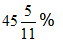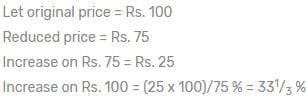CLAT  >  Test: Percentage- 1

# Test: Percentage- 1

Test Description

## 20 Questions MCQ Test Quantitative Techniques for CLAT | Test: Percentage- 1

Test: Percentage- 1 for CLAT 2023 is part of Quantitative Techniques for CLAT preparation. The Test: Percentage- 1 questions and answers have been prepared according to the CLAT exam syllabus.The Test: Percentage- 1 MCQs are made for CLAT 2023 Exam. Find important definitions, questions, notes, meanings, examples, exercises, MCQs and online tests for Test: Percentage- 1 below.
Solutions of Test: Percentage- 1 questions in English are available as part of our Quantitative Techniques for CLAT for CLAT & Test: Percentage- 1 solutions in Hindi for Quantitative Techniques for CLAT course. Download more important topics, notes, lectures and mock test series for CLAT Exam by signing up for free. Attempt Test: Percentage- 1 | 20 questions in 30 minutes | Mock test for CLAT preparation | Free important questions MCQ to study Quantitative Techniques for CLAT for CLAT Exam | Download free PDF with solutions
 1 Crore+ students have signed up on EduRev. Have you?
Test: Percentage- 1 - Question 1

### A batsman scored 110 runs which included 3 boundaries and 8 sixes. What percent of his total score did he make by running between the wickets?

Detailed Solution for Test: Percentage- 1 - Question 1

Number of runs made by running:-

110- (3*4+8*6)

= 110- 60

= 50

Therefore, required percentage :-

[( 50/ 110)  * 100] %

=>Test: Percentage- 1 - Question 2

### If two numbers are respectively 20% and 50% more than the 3rd number then what % is the 1st number of the 2nd one?

Detailed Solution for Test: Percentage- 1 - Question 2

Two numbers are respectively 20% and 50% more than the 3rd number. What percent is the first of the second? Consider 3rd number be 100%.

Then percent of 1st number to 2nd number =(120%/150%)×100=80%

Test: Percentage- 1 - Question 3

### If the radius of circle is increased by 110% its area is increased by

Detailed Solution for Test: Percentage- 1 - Question 3

Option ( c) 341 is correct.

Explanation:-

{Let the initial radius of circle = 10 cm

Then, it's area = π r^ 2

=  π (10) ^2

=  100 π cm square.

Given, (radius is  increased by 110%)

New radius = ( 100+110) % of 10 cm

=> 210% of 10 cm

{ 210 /100 * 10} cm

=> 21 cm

Therefore, area of circle of radius (21) cm

=> π (21) ^2

=>  441 π cm square.

Increase in area=  ( 441 π - 100 π ) cm square.
=> 341 cm square. }

Test: Percentage- 1 - Question 4

In an election between two candidates, one got 55% of the total valid votes, 20% of the votes were invalid. If the total number of votes was 7500, the number of valid votes that the other candidate got, was:

Detailed Solution for Test: Percentage- 1 - Question 4

= 80% of 7500
= 6000
∴ Valid votes polled by other candidate
= 45% of 6000
=  45100×600045100×6000 = 2700

Test: Percentage- 1 - Question 5

In an exam 80% of the students passed in Eng, 85% in Math and 75% in both. if 40 students failed in both subjects, the total number of students is?

Detailed Solution for Test: Percentage- 1 - Question 5

Let n(A) = no. of students pass in english
n(B) = no. of students pass in math
n(A U B) = no. of students pass in either math or english
n(A ∩ B) = no.of students pass in both math and english
let x are no. of students
⇒ n(A U B) = n(A) + n(B) - n(A ∩ B)
= 80x/100 + 85x/100 - 75x/100
= 90x/100
no. of students fail in both either math or english = x - n(A U B)

⇒ 40 = x - 90x/100

⇒ 40 =  x/10

⇒  x = 400
total no. of students = 400

Test: Percentage- 1 - Question 6

Mrs. Ruhani travels with 80% of her usual speed and thus gets late by 40 minutes. What will be the usual time taken by her in covering the same distances?

Test: Percentage- 1 - Question 7

The price of rice is increased by 25% and as a result, a person can have 4kg less rice for Rs.400. What is the increase price of rice per kg?

Test: Percentage- 1 - Question 8

In an examination, Summit got 30% marks and failed by 15 marks. In the same examination, Rohit got 40% marks, which is 35 marks more than the pass marks. Then what is the total marks in the examination?

Detailed Solution for Test: Percentage- 1 - Question 8

The correct option is A.
Let the total marks be x.
Sumit got = 30% of x
= 0.3x
Passing marks = 0.3x+15

Rohit got = 40% of x
= 0.4x
Passing marks = 0.4x - 35

Equating both passing marks , we get
0.3x+15 = 0.4x - 35
50 = 0.1x
x = 500

Test: Percentage- 1 - Question 9

After spending 40% in machinery, 25% in building, 15% in raw material and 5% in furniture, Harilal had a balance of Rs. 1305. The money with him was

Test: Percentage- 1 - Question 10

In Mathematics exam, a student scored 30% marks in the first paper, out of the total of 180. How much should he score in second paper out of a total of 150, if he is to get an overall average of at least 50%

Detailed Solution for Test: Percentage- 1 - Question 10

Firstly, marks he scored in first paper out of 180 will be

180 x 30÷100 = 54 marks

So he scored 54 marks out of 180 in the first paper.

Now total marks of the examination is 330 i.e 150+180.

50% of 330 is 165.

So in second paper he has to score 165–54=111 marks to get 50% marks over all.

Now the ans is asked in terms of percent so,

111÷150 x 100 = 74%

So he has to get 74 % in the second paper to get a overall percent of 50%.

Test: Percentage- 1 - Question 11

In an examination, it is required to get 36% of maximum marks to pass. A student got 113 marks and was declared fail by 85 marks. The maximum marks are

Detailed Solution for Test: Percentage- 1 - Question 11

The correct option is B.
Let maximum marks be x.
Then
36% of x = (113+85). So x = (198*100/ 36) = 550

Test: Percentage- 1 - Question 12

In an examination, 42% students failed in Hindi and 52% failed in English. If 17% failed in both the subjects, the percentage of those who passed in both the subject is

Detailed Solution for Test: Percentage- 1 - Question 12 It is given that 42% fail in Hindi and 52% fail in English and 17% fail in both
So total fail percentage would be
42+52-17 ( because we have counted the 17 % 2 times)
=> 77 % who have failed SO the percentage passing in both would be
100-77= 23%
Test: Percentage- 1 - Question 13

In a college election, a candidate secured 62% of the votes and is elected by a majority of 144 votes. The total number of votes polled is

Detailed Solution for Test: Percentage- 1 - Question 13
∵ (62% of N - 38% of N) =144
⇒ 24% of N = 144
∴ N = (144 x 100)/24 = 600
Test: Percentage- 1 - Question 14

The price of sugar is increased by 20%. If the expenditure is not allowed to be increased, the ratio between the reduction in consumption and the original consumption is

Test: Percentage- 1 - Question 15

On decreasing the price of TV set by 30%, its sale is increased by 20%. What is the effect on the revenue received by the shopkeeper?

Detailed Solution for Test: Percentage- 1 - Question 15
Lets take the orignal price as 100. Then on decreasing the price by 30% we get= 70. Now the sale is increased by 20%=84 (20% of 70 or 20/100*70= 14 now 70+14=84) So we can say that Cp=100 and Sp=84 Thats loss as cp is bigger. Loss=16 Loss%=16/100*100 =16%

Test: Percentage- 1 - Question 16

The value of a machine depreciates 10% annually. If its present value is Rs. 4000, its value 2 years hence will be:

Detailed Solution for Test: Percentage- 1 - Question 16

The correct option is B.
Present value - 4000
Depreciation - 10% of present value annually
After one year value of machine will be =  4000 - 400(10% of 4000) = 3600
After 2nd year value of machine will be = 3600 - 360(10% of 3600) = 3240

Test: Percentage- 1 - Question 17

The population of a town increases by 5% annually. If it is 15435 now, its population 2 years ago was:

Test: Percentage- 1 - Question 18

A man’s wages were decreased by 50%. Again the reduced wages were increased by 50%. He has a loss of:

Test: Percentage- 1 - Question 19

A number when increased by 37 ½% gives 33. The number is:

Test: Percentage- 1 - Question 20

The price of an article has been reduced to 25%. In order to restore the original price, the new price must be increased by:

Detailed Solution for Test: Percentage- 1 - Question 20## Quantitative Techniques for CLAT

56 videos|35 docs|91 tests
 Use Code STAYHOME200 and get INR 200 additional OFF Use Coupon Code
Information about Test: Percentage- 1 Page
In this test you can find the Exam questions for Test: Percentage- 1 solved & explained in the simplest way possible. Besides giving Questions and answers for Test: Percentage- 1, EduRev gives you an ample number of Online tests for practice

## Quantitative Techniques for CLAT

56 videos|35 docs|91 tests